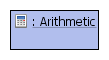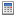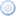#Arithmetic extendsActivityNode, DataFlowEdgeDestination, DataFlowEdgesSource

An inline expression of arithmetic, operating upon all incoming values.

An must have at least one incoming , representing the source values. When a sourced from a is evaluated, the value provided represents the arithmetic combination of the source values according to the of the .

Four are defined for : `AND`, `SUBTRACT`, `MULTIPLY` and `DIVIDE`; each of these operations correspond to addition, subtraction, multiplication and division, respectively. For `DIVIDE` arithmetic, the divisor used in the arithmetic is the first incoming with the "divisor". If the divisor used in a `DIVIDE` arithmetic is equal to zero, the value of the subsequent node will not be numeric ("not-a-number", or `NaN`).

This model element may be removed from the IAML metamodel in the future, as these arithmetic operations can be replaced with .

## Examples

• A summary of all of the elements used in .

## Implementation Notes

• can be used inline to add together itsincoming operands, and act as a source of data.
• can be used inline to divide itsincoming operands, and act as a source of data.
• can be used inline to multiply itsincoming operands, and act as a source of data.
• can be used inline to subtract itsincoming operands, and act as a source of data.
• If is used to divide by zero, `NaN` willresult.

(none)

## Constraints

• "Arithmetic must have at least one incoming data source edge"

## Attributes

• operationType : ArithmeticOperationTypes (optional)
The builtin arithmetic operation to perform.
Accepted literals: ADD, SUBTRACT, MULTIPLY, DIVIDE

## Inherited Attributes

• generatedRule : EString (optional)
To assist in debugging, this attribute may be populated with the name of the model completion rule that created this element. More extensive traceability support may be provided in the future.
• id : EString (optional)
A unique identifier for this model element. Should be globally unique.
• isGenerated : EBoolean (optional)
Set to `true` if the current element has been generated.

(none)

(none)

(none)

## Inherited References

• generatedBy : [0...*] [opposite: generatedElements]
A list of that generated this element; conversely, the source will prevent from being created.
• inFlows : [0...*] [opposite: to]
A list of incoming .
• outFlows : [0...*] [opposite: from]
A list of outgoing .

(none)